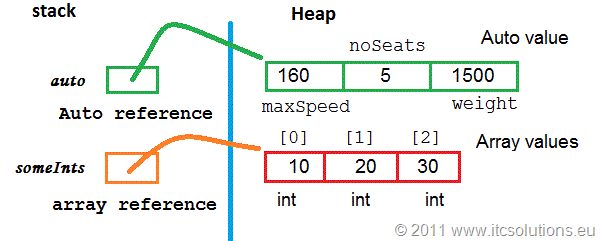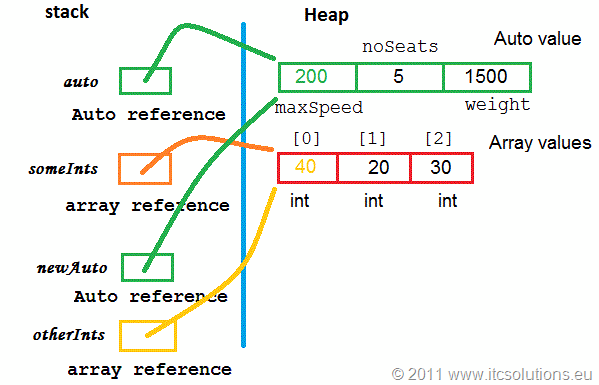# Tutorial Java – #7 Reference data type variables

In this post it is described one of the most important concepts of Object Oriented Programming: Every class instance, object, is managed only by reference type variables (for those who know also C++, you could remember that in C++ this rule is not entirely true because objects are also managed by value type variables).

This rule is very important to understand because it’s a fundamental fact on which Object Oriented Programming languages (also C#) have been defined. Understanding it, will help you understand and learn Java programming.

Other topics that are part of this Java tutorial are accessible through Java 6 Tutorial – Contents.

## What are reference data type variables

Reference variables, or references, are variables (like a primitive data type, let’s say int vb) because they require memory space to store their values. The main difference between a reference and primitive variable is that the values for the first one are numbers that represent addresses, mainly, of memory zones in Heap.

When a process is started (your application) it needs active memory (Random Access Memory) to store its data and also its code and stack (a fixed temporary buffer for future use). The JVM (Java Virtual Machine) reserves memory space for it based on what it can determine before run-time (based on variables defined in the source code) and during the application execution.

So the process manages and needs two data memory zones:

• stack – the fixed size one; its size is determined based on information available before the execution of a function (remember that the most simple running Java application requires one function – main); this information is given by the function local and input variables (for main, its local variables are in fact the application variables);
• Heap – the dynamic one; it represents the memory requirements for data that is needed or not at the runtime; you don’t know for sure if you need it and which is going to be its size, because it depends on the application execution and other external input (let’s suppose we have an application that records the values of an array, but its size and values are given during run-time by the user; so you know the application will need some extra memory space but you don’t know how many bytes because you don’t know what the user will insert); in order to reserve this space, at run-time, we will use the new operator or function; the Heap is also known as a dynamic managed memory because it responds to memory requests from different processes and also release used memory when it no more needed (more on this in the Garbage Collector post).

Here is an example that generates an array with a length given at run-time by the user:

```        try
{
int[] intValues;
int noElements = 0;
System.out.println("The array elements number (0 - 255):");

intValues = new int[noElements];

//print the values - all with 0 default value
for(int i=0; i < intValues.length; i++)
System.out.print(" "+intValues[i]);

}
catch(IOException ex){
System.out.println(ex.getMessage());
}```

The general rule regarding where variables are put in memory is:

• variables declared in functions blocks (in Java you CAN’T declare global variables like in C or C++) or in their parameters list are placed on the stack (function stack);
• any value created with new operator is placed on the Heap.

So, the previous code sequence (do not forget that in Java any array is an object –> the array reference is on the stack and its values are in Heap) generates this memory imageObjects value and references in memory

## How to define reference data type variables

The syntax for defining references is:

class_name reference_name;

Every time you define a reference, it has a default value equal to null IF it is not a local variable (a predefined value indicating no address; in C/C++ the null value is equivalent to 0). This means that it can’t be used.

If the reference is a local variable (and NOT a instance variable – attribute) then you will receive a compiler error.

If we consider this class

```class Auto{
int maxSpeed;
float weight;
int noSeats;
}```

and test it, we get a variable auto might not have been initialized compiler error if we try this

```public class TestAuto {
public static void main(String[] args) {
//define local variable/reference
//local variables are not initialized with a default
Auto auto;          //compiler error
auto.maxSpeed = 160;
}
}```

or we get a java.lang.NullPointerException run-time exception for this (because the object does not exists and there is no memory space where to store 160 value):

```public class TestAuto {
public static void main(String[] args) {
//define local variable/reference
//init it with the default null value
Auto auto = null;
auto.maxSpeed = 160;    //run-time exception
}
}```

## How to initialize reference data type variables

As I said, all references are variables that have addresses (numbers) as values. In order to get an address we must request and reserve some memory in Heap (only here). And, this is done with the new operator (also used in Tutorial Java 6 – #4 Arrays and Tutorial Java – #6 Classes and objects) like this:

reference_type reference = new  reference_type(); //for objects

base_type array_reference = new base_type[elements_number]  //for arrays

If we consider this Java sample:

```public class TestAuto {
public static void main(String[] args) {
//define an Auto reference
//init it
Auto auto = new Auto();
//init object values
auto.maxSpeed = 160;
auto.noSeats = 5;
auto.weight = 1500;

//define and init an array reference
int[] someInts = new int;
someInts = 10;
someInts = 20;
someInts = 30;
}
}```

then it will look in memory like this:Memory image of objects value and their references

Attention ! When you use = (equal) between two references, the value of the right reference is copied into the left reference. It is important to understand that what is copied it is the reference value and not the object or array value which is in Heap. This type of copy is know as shallow copy.

For example, if we add some instructions to the initial situation (previous example)

```        Auto newAuto = auto;
int[] otherInts = someInts;```Object values managed by two references

in memory we will have 4 references on the main function stack and only 2 values (one object and one array) in Heap. In this case, we have access to the object value through two different references that have the same value:

```        newAuto.maxSpeed = 200; //same result as auto.maxSpeed = 200;
System.out.println(auto.maxSpeed);  //prints 200
otherInts = 40;  //same result as someInts = 40;
System.out.println(someInts);  //prints 40```

Other topics that are part of this Java tutorial are accessible through Java 6 Tutorial – Contents.